# Recursion You will learn what is recursion as

• Slides: 32Recursion You will learn what is recursion as well as how and when to use it in your programs. James TamWhat Is Recursion? “the determination of a succession of elements by operation on one or more preceding elements according to a rule or formula involving a finite number of steps” (Merriam. Webster online) James Tam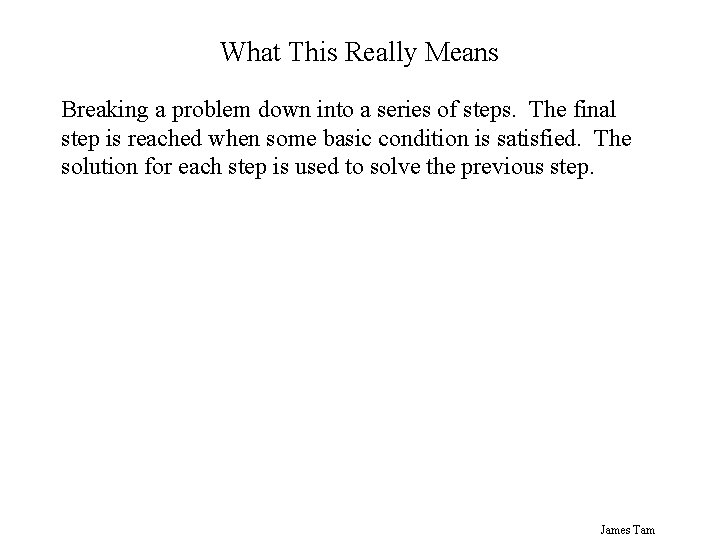What This Really Means Breaking a problem down into a series of steps. The final step is reached when some basic condition is satisfied. The solution for each step is used to solve the previous step. James Tam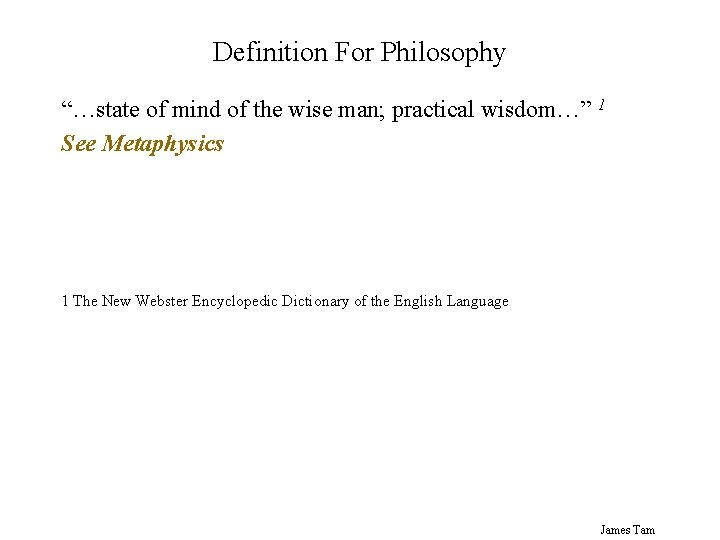Definition For Philosophy “…state of mind of the wise man; practical wisdom…” 1 See Metaphysics 1 The New Webster Encyclopedic Dictionary of the English Language James Tam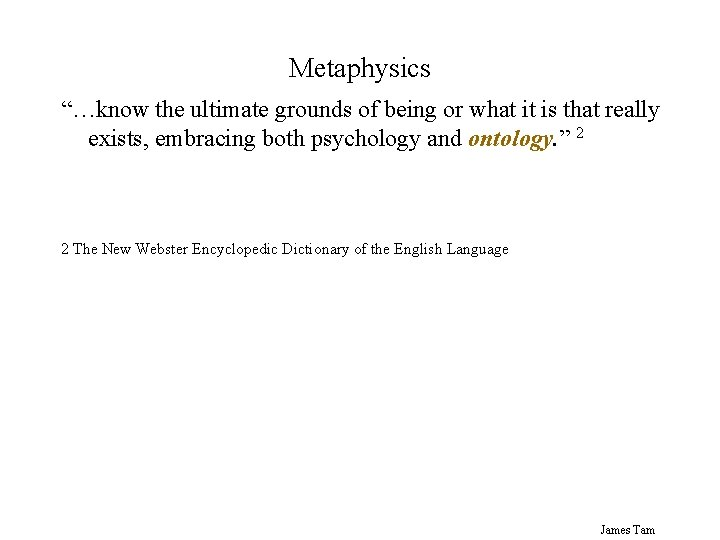Metaphysics “…know the ultimate grounds of being or what it is that really exists, embracing both psychology and ontology. ” 2 2 The New Webster Encyclopedic Dictionary of the English Language James Tam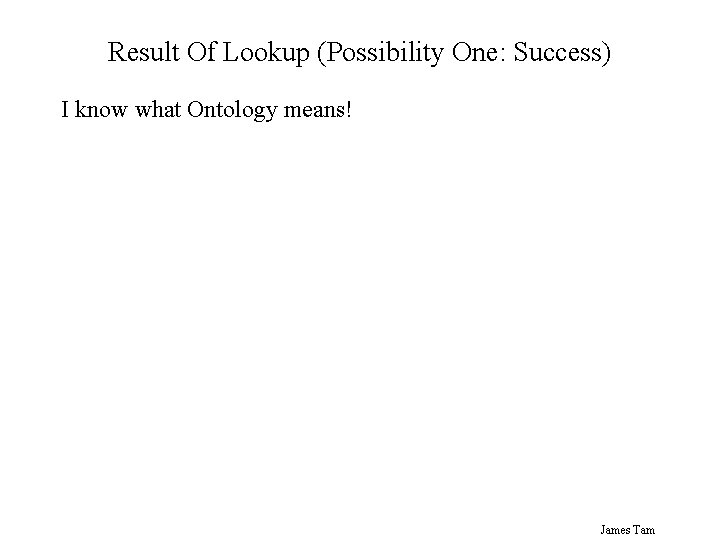Result Of Lookup (Possibility One: Success) I know what Ontology means! James Tam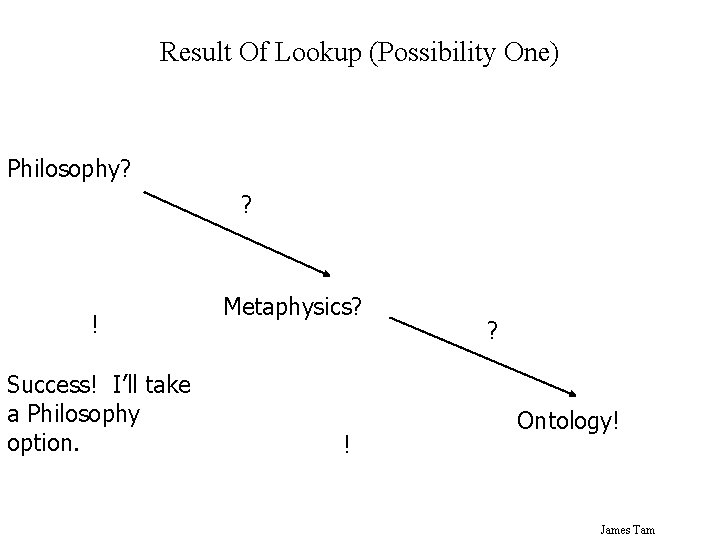Result Of Lookup (Possibility One) Philosophy? ? ! Success! I’ll take a Philosophy option. Metaphysics? ! ? Ontology! James Tam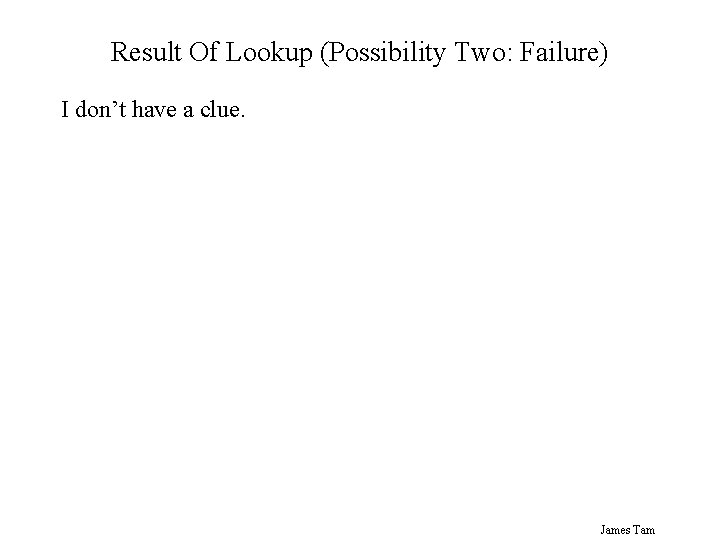Result Of Lookup (Possibility Two: Failure) I don’t have a clue. James TamResult Of Lookup (Possibility Two) Philosophy? ? Metaphysics? ? Rats! I’m taking MGIS instead. ? Ontology? James TamOntology “…equivalent to metaphysics. ” 3 3 The New Webster Encyclopedic Dictionary of the English Language James Tam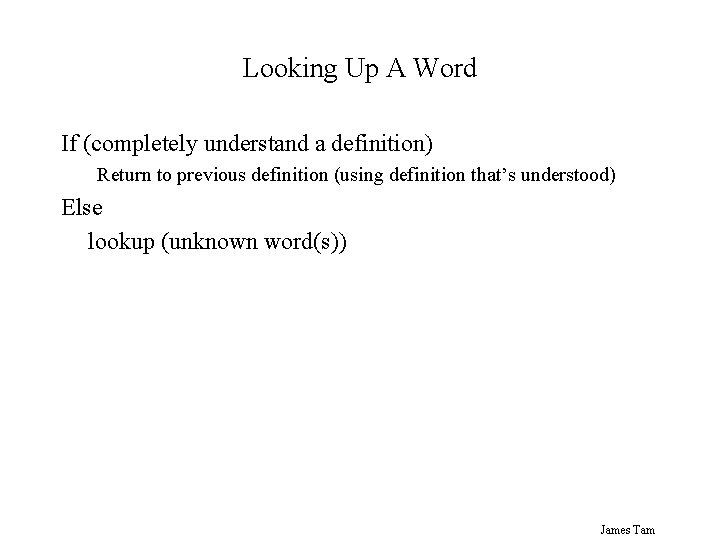Looking Up A Word If (completely understand a definition) Return to previous definition (using definition that’s understood) Else lookup (unknown word(s)) James Tam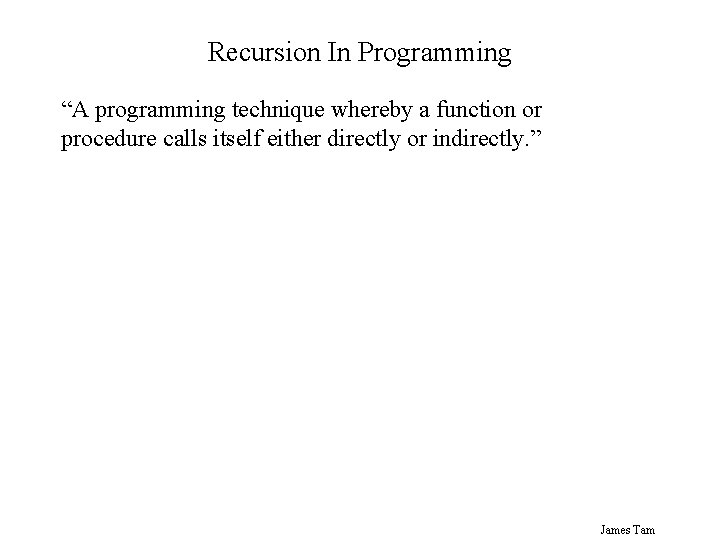Recursion In Programming “A programming technique whereby a function or procedure calls itself either directly or indirectly. ” James TamDirect Call function James TamIndirect Call fun 1 fun 2 fun 3 … no. More. Fun James Tam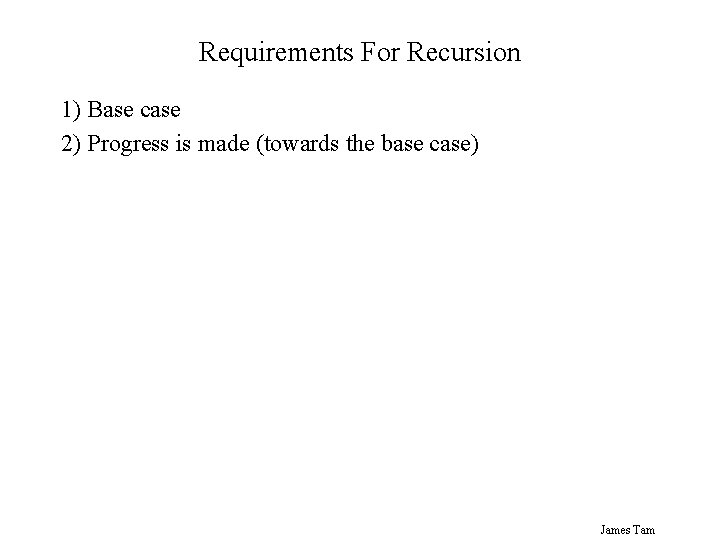Requirements For Recursion 1) Base case 2) Progress is made (towards the base case) James Tam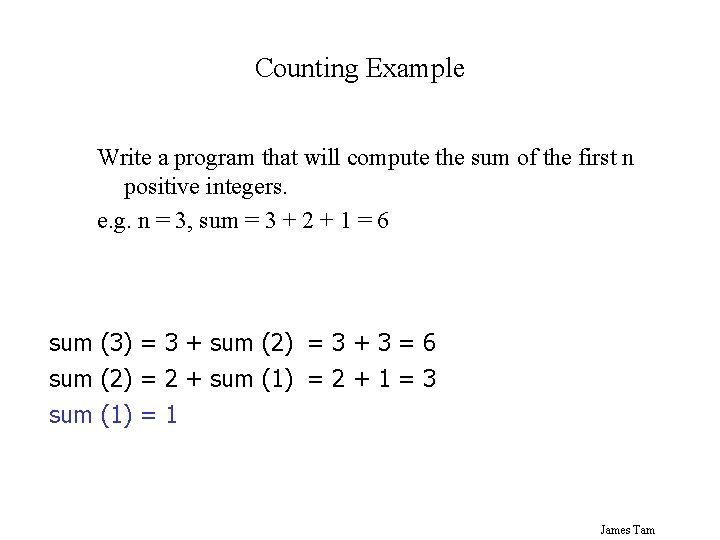Counting Example Write a program that will compute the sum of the first n positive integers. e. g. n = 3, sum = 3 + 2 + 1 = 6 sum (3) = 3 + sum (2) = 3 + 3 = 6 sum (2) = 2 + sum (1) = 2 + 1 = 3 sum (1) = 1 James TamExample Program program sum. Series (input, output); var last. Number : integer; total : integer; function sum (no : integer): integer; begin if (no = 1) then sum : = 1 else sum: = (no + sum (no - 1)); end; begin write('Enter the last number in the series : '); readln(last. Number); total : = sum(last. Number); writeln('Sum of the series from 1 - ', last. Number, ' is, ', total); end. sum. Series 6 total = sum(3) sum (3) if (3 = 1) then F sum : = 1 else 3 sum : = (3 +sum (3 – 1)); sum (2) if (2 = 1) then F sum : = 1 else 1 sum : = (2 +sum (2 – 1)); sum (1) if (1 = 1) then T sum : = 1 James TamIndirect Recursion In Pascal For full example look under /home/231/examples/functions/indirect. p Example Scenario: procedure proc 1 calls procedure proc 2 but procedure proc 2 calls procedure proc 1 Which one comes first? James Tam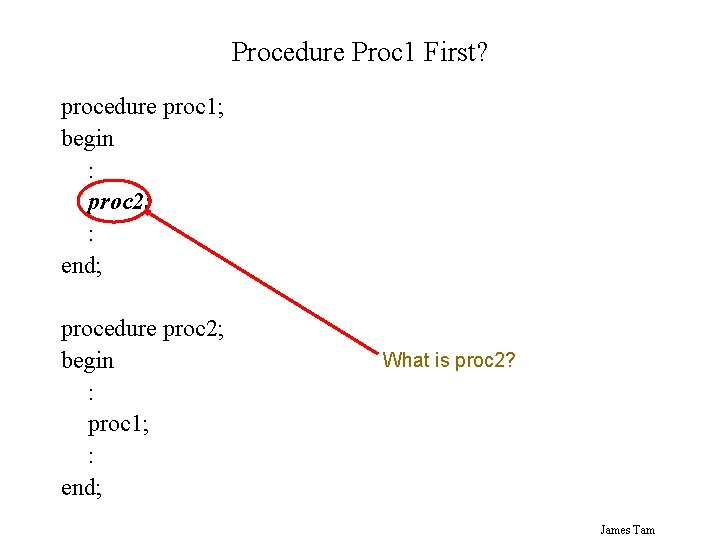Procedure Proc 1 First? procedure proc 1; begin : proc 2; : end; procedure proc 2; begin : proc 1; : end; What is proc 2? James TamProcedure Proc 2 First? procedure proc 2; begin : proc 1; : end; procedure proc 1; begin : proc 2; : end; What is proc 1? James TamSolution: Use A Dummy Definition A "placeholder" for the compiler (definition comes later) Example problem procedure proc 1; begin : proc 2; : end; procedure proc 2; begin : proc 1; : end; James Tam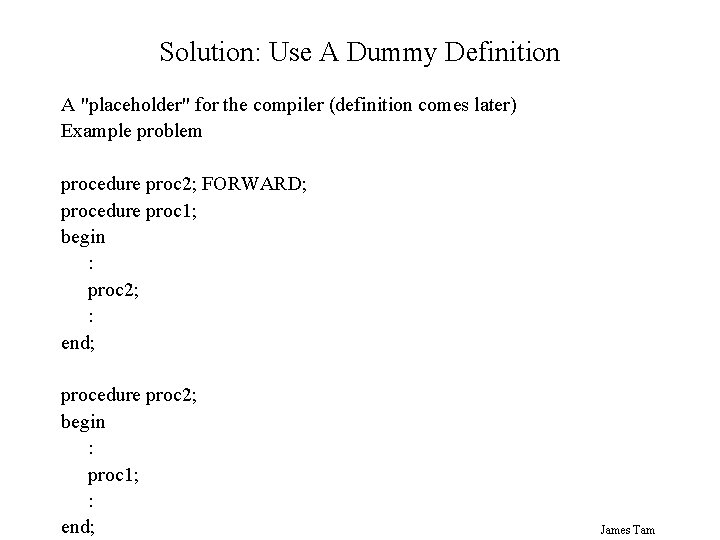Solution: Use A Dummy Definition A "placeholder" for the compiler (definition comes later) Example problem procedure proc 2; FORWARD; procedure proc 1; begin : proc 2; : end; procedure proc 2; begin : proc 1; : end; James TamWhen To Use Recursion When a problem can be divided into steps The result of one step can be used in a previous step All of the results together solve the problem James TamWhen To Consider Alternatives To Recursion When a loop will solve the problem just as well James Tam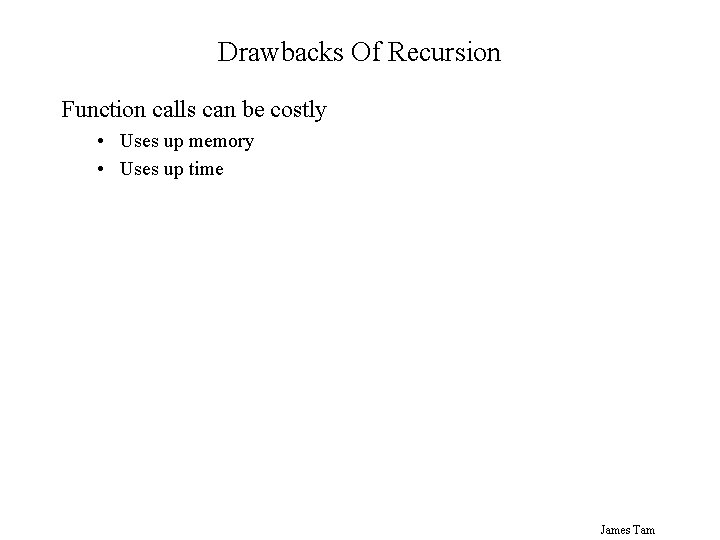Drawbacks Of Recursion Function calls can be costly • Uses up memory • Uses up time James TamBenefits Of Using Recursion Simpler solution that’s more elegant (for some problems) Easier to visualize solutions (for some people) James TamCommon Pitfalls When Using Recursion No base case No progress towards the base case Using up too many resources (e. g. , variable declarations) for each function call James Tam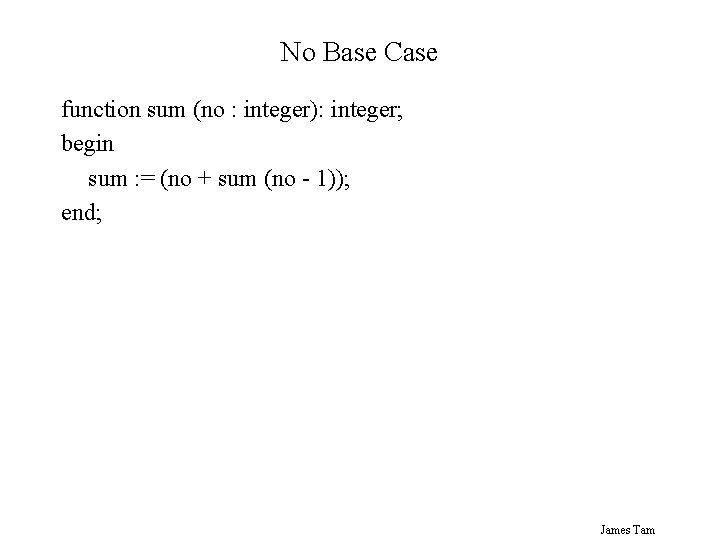No Base Case function sum (no : integer): integer; begin sum : = (no + sum (no - 1)); end; James TamNo Progress Towards Base Case function sum (no : integer): integer; begin if (no = 1) then sum : = 1 else sum : = (no + sum (no)); end; James Tam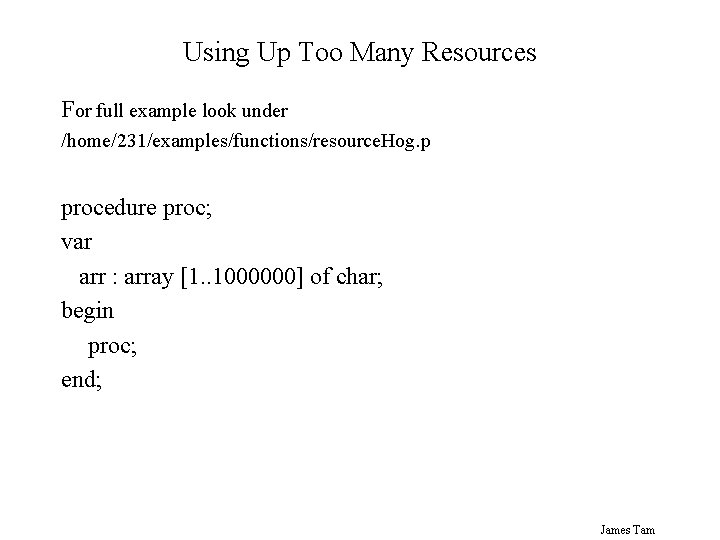Using Up Too Many Resources For full example look under /home/231/examples/functions/resource. Hog. p procedure proc; var arr : array [1. . 1000000] of char; begin proc; end; James Tam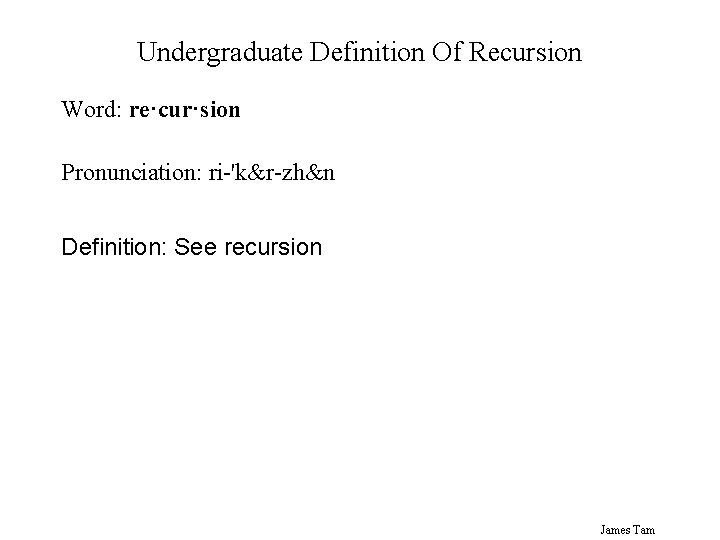Undergraduate Definition Of Recursion Word: re·cur·sion Pronunciation: ri-'k&r-zh&n Definition: See recursion James TamSummary Description of recursion Real world example Trace of a recursive Pascal program Benefits and drawbacks of using recursion When to use recursion and when to consider alternatives What are the potential pitfalls of using recursion Alternative definition of recursion James Tam